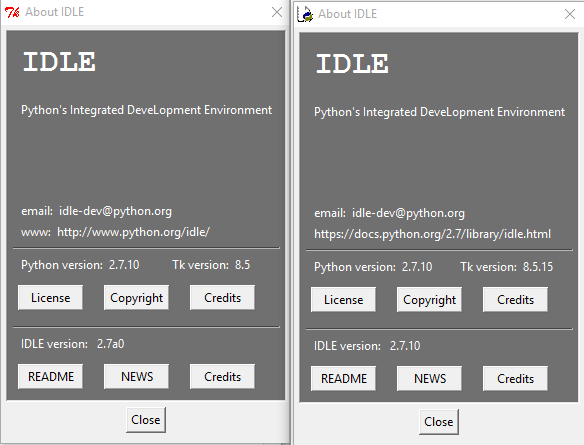# Zumo arduino UNO and minIMU-9

Hi.

I’ve followed the steps of installing every python program needed here:https://github.com/pololu/minimu-9-ahrs-arduino

I’ve got windows 10

I upload de .ino program to the arduino and i receive the ang from serial port.

I change the port on MinIMU-9-test.py but when i run it, i see the angles on Python Shell are being receive but it doesn’t draw anything. The windows are grey and they not respond.

Any help? Thanks!

Hello, Drazz.

I’ve moved your topic to a more appropriate section of the forum.

I tried setting up Python and the visualization program on a Windows 10 computer here, and it worked fine. Which version of Python you have installed? If it is not Python 2.7.10, could you try installing that version (the direct link is python.org/ftp/python/2.7.1 … 2.7.10.msi ) and reinstalling the extensions (also from the direct links we provide)?

- Kevin

Hello Kevin!

This is my version of Python. I think is correct.If i change line `while(1):`

to

``````...
t=0
while t<1000:
t=t+1
... [/code]

I get the correct answer at the end of the simulation!

Just in case, i send you the code (i've only modifed that and the COM port, rest is the same)

[code]# MinIMU9ArduinoAHRS
# Pololu MinIMU-9 + Arduino AHRS (Attitude and Heading Reference System)

# Copyright (c) 2011 Pololu Corporation.
# https://www.pololu.com/

# MinIMU9ArduinoAHRS is based on sf9domahrs by Doug Weibel and Jose Julio:

# sf9domahrs is based on ArduIMU v1.5 by Jordi Munoz and William Premerlani, Jose
# Julio and Doug Weibel:

# MinIMU9ArduinoAHRS is free software: you can redistribute it and/or modify it
# Free Software Foundation, either version 3 of the License, or (at your option)
# any later version.

# MinIMU9ArduinoAHRS is distributed in the hope that it will be useful, but
# WITHOUT ANY WARRANTY; without even the implied warranty of MERCHANTABILITY or
# FITNESS FOR A PARTICULAR PURPOSE. See the GNU Lesser General Public License for
# more details.

# You should have received a copy of the GNU Lesser General Public License along
# with MinIMU9ArduinoAHRS. If not, see <http://www.gnu.org/licenses/>.

################################################################################

# This is a test/3D visualization program for the Pololu MinIMU-9 + Arduino
# AHRS, based on "Test for Razor 9DOF IMU" by Jose Julio, copyright 2009.

# This script needs VPython, pyserial and pywin modules

# First Install Python 2.6.4 (Python 2.7 also works)
# Install pywin from http://sourceforge.net/projects/pywin32/
# Install pyserial from http://sourceforge.net/projects/pyserial/files/

from visual import *
import serial
import string
import math

from time import time

# Check your COM port and baud rate
ser = serial.Serial(port='COM4',baudrate=115200, timeout=1)

# Main scene
scene=display(title="Pololu MinIMU-9 + Arduino AHRS")
scene.range=(1.2,1.2,1.2)
#scene.forward = (0,-1,-0.25)
scene.forward = (1,0,-0.25)
scene.up=(0,0,1)

# Second scene (Roll, Pitch, Yaw)
scene2 = display(title='Pololu MinIMU-9 + Arduino AHRS',x=0, y=0, width=500, height=200,center=(0,0,0), background=(0,0,0))
scene2.range=(1,1,1)
scene.width=500
scene.y=200

scene2.select()
#Roll, Pitch, Yaw
arrow_course = arrow(pos=(0.6,0,0),color=color.cyan,axis=(-0.2,0,0), shaftwidth=0.02, fixedwidth=1)

#Roll,Pitch,Yaw labels
label(pos=(-0.4,0.3,0),text="Roll",box=0,opacity=0)
label(pos=(0.1,0.3,0),text="Pitch",box=0,opacity=0)
label(pos=(0.55,0.3,0),text="Yaw",box=0,opacity=0)
label(pos=(0.6,0.22,0),text="N",box=0,opacity=0,color=color.yellow)
label(pos=(0.6,-0.22,0),text="S",box=0,opacity=0,color=color.yellow)
label(pos=(0.38,0,0),text="W",box=0,opacity=0,color=color.yellow)
label(pos=(0.82,0,0),text="E",box=0,opacity=0,color=color.yellow)
label(pos=(0.75,0.15,0),height=7,text="NE",box=0,color=color.yellow)
label(pos=(0.45,0.15,0),height=7,text="NW",box=0,color=color.yellow)
label(pos=(0.75,-0.15,0),height=7,text="SE",box=0,color=color.yellow)
label(pos=(0.45,-0.15,0),height=7,text="SW",box=0,color=color.yellow)

L1 = label(pos=(-0.4,0.22,0),text="-",box=0,opacity=0)
L2 = label(pos=(0.1,0.22,0),text="-",box=0,opacity=0)
L3 = label(pos=(0.7,0.3,0),text="-",box=0,opacity=0)

# Main scene objects
scene.select()
# Reference axis (x,y,z)
arrow(color=color.green,axis=(1,0,0), shaftwidth=0.02, fixedwidth=1)
arrow(color=color.green,axis=(0,-1,0), shaftwidth=0.02 , fixedwidth=1)
arrow(color=color.green,axis=(0,0,-1), shaftwidth=0.02, fixedwidth=1)
# labels
label(pos=(0,0,0.8),text="Pololu MinIMU-9 + Arduino AHRS",box=0,opacity=0)
label(pos=(1,0,0),text="X",box=0,opacity=0)
label(pos=(0,-1,0),text="Y",box=0,opacity=0)
label(pos=(0,0,-1),text="Z",box=0,opacity=0)
# IMU object
platform = box(length=1, height=0.05, width=1, color=color.blue)
p_line = box(length=1,height=0.08,width=0.1,color=color.yellow)
plat_arrow = arrow(color=color.green,axis=(1,0,0), shaftwidth=0.06, fixedwidth=1)

f = open("Serial"+str(time())+".txt", 'w')

roll=0
pitch=0
yaw=0
while (1):
if line.find("!ANG:") != -1:          # filter out incomplete (invalid) lines
line = line.replace("!ANG:","")   # Delete "!ANG:"
print line
f.write(line)                     # Write to the output log file
words = string.split(line,",")    # Fields split
if len(words) > 2:
try:
except:
print "Invalid line"

axis=(cos(pitch)*cos(yaw),-cos(pitch)*sin(yaw),sin(pitch))
up=(sin(roll)*sin(yaw)+cos(roll)*sin(pitch)*cos(yaw),sin(roll)*cos(yaw)-cos(roll)*sin(pitch)*sin(yaw),-cos(roll)*cos(pitch))
platform.axis=axis
platform.up=up
platform.length=1.0
platform.width=0.65
plat_arrow.axis=axis
plat_arrow.up=up
plat_arrow.length=0.8
p_line.axis=axis
p_line.up=up
cil_roll.axis=(0.2*cos(roll),0.2*sin(roll),0)
cil_roll2.axis=(-0.2*cos(roll),-0.2*sin(roll),0)
cil_pitch.axis=(0.2*cos(pitch),0.2*sin(pitch),0)
cil_pitch2.axis=(-0.2*cos(pitch),-0.2*sin(pitch),0)
arrow_course.axis=(0.2*sin(yaw),0.2*cos(yaw),0)
L1.text = str(float(words))
L2.text = str(float(words))
L3.text = str(float(words))
ser.close
f.close``````

Thanks a lot

Hi, Drazz.

I looked at your problem some more and realized it looks very similar to this issue I saw a few years ago. Specifically, VPython 6 does not work well with the AHRS program. Did you make sure to install the recommended version of VPython (5.74)? The direct link to that version is:

Solved. Thanks a lot!